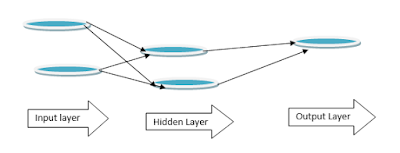## Wednesday, 13 September 2017

### Neural Network: Forward Propagation in PythonStructure of Neural Network

Here is your first forward propagation Algorithm in python-

import numpy as np
inputs= np.array([1,2])
weight0 =np.array([1,-1])
weight1 = np.array([1,2])
weight2= np.array([2,-1])
hiddenValue1 = (inputs*weight0).sum()
hiddenValue2 = (inputs*weight1).sum()
hiddenlayer_val =np.array([hiddenValue1,hiddenValue2])
output_val=(hiddenlayer_val*weight2).sum()
print(output_val)

1)      Use of Activation functions- to include non-linearity. An "activation function" is a function applied at each node. It converts the node's input into some output. Ex-

ReLu ( Rectified Linear Activation)ReLu Activation Function
Here is your first forward propagation Algorithm with Activation function in python-

import numpy as np
def relu(input) :
op=max(input,0)
return(op)

inputs= np.array([1,2])
weight0 =np.array([1,-1])
weight1 = np.array([1,2])
weight2= np.array([2,-1])
node0_input <- (input*weight0).sum()
node1_input <- (input* weight1).sum()
node0_output <-relu(node0_input)
node1_output <- relu(node1_input)
hidden_layer_output= np.array(node0_output, node1_output)
output <- (hidden_layer_output*weights2).sum()
print(output)

ABC of deep Learning